# Data Mining and machine learning The A Priori

• Slides: 45Data Mining (and machine learning) The A Priori Algorithm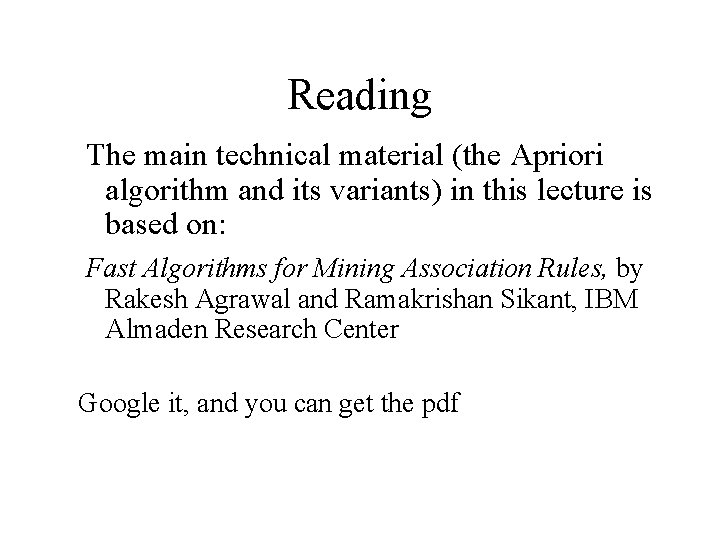Reading The main technical material (the Apriori algorithm and its variants) in this lecture is based on: Fast Algorithms for Mining Association Rules, by Rakesh Agrawal and Ramakrishan Sikant, IBM Almaden Research Center Google it, and you can get the pdf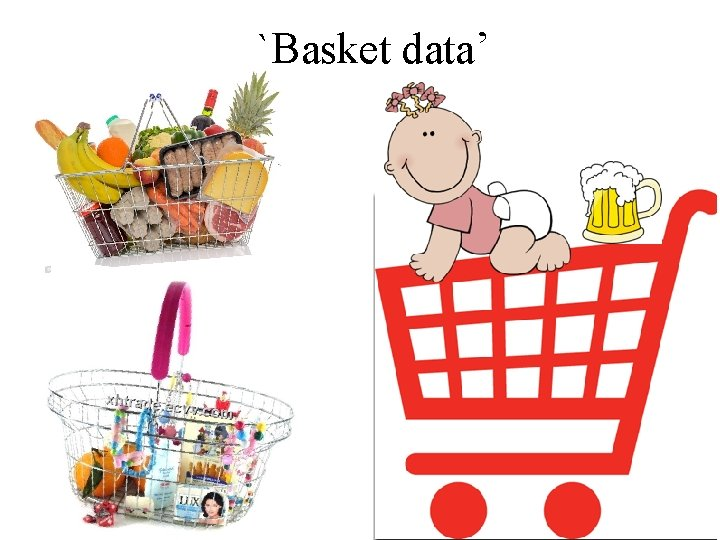`Basket data’ A very common type of data; often also called transaction data. Next slide shows example transaction database, where each record represents a transaction between (usually) a customer and a shop. Each record in a supermarket’s transaction DB, for example, corresponds to a basket of specific items.ID 1 2 3 4 5 6 7 8 9 10 11 12 13 14 15 16 17 18 19 20 apples, beer, cheese, dates, eggs, 1 1 1 1 fish, glue, 1 honey, ice-cream 1 1 1 1 1 1 1 1 1 1 1Numbers Our example transaction DB has 20 records of supermarket transactions, from a supermarket that only sells 9 things One month in a large supermarket with five stores spread around a reasonably sized city might easily yield a DB of 20, 000 baskets, each containing a set of products from a pool of around 1, 000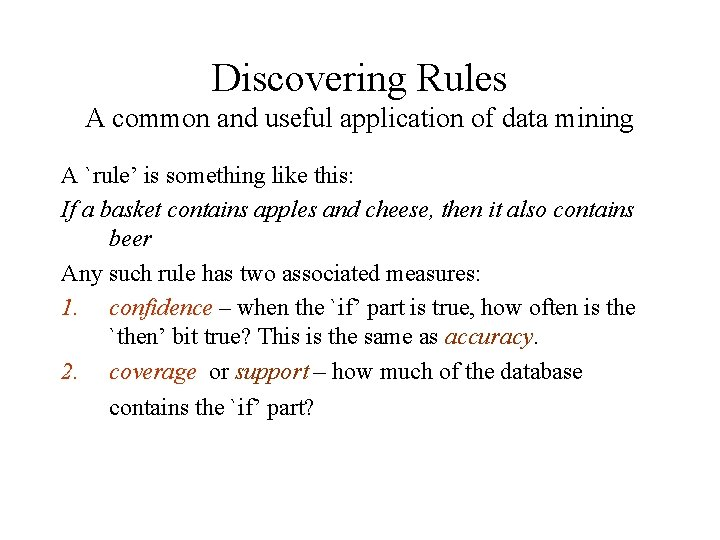Discovering Rules A common and useful application of data mining A `rule’ is something like this: If a basket contains apples and cheese, then it also contains beer Any such rule has two associated measures: 1. confidence – when the `if’ part is true, how often is the `then’ bit true? This is the same as accuracy. 2. coverage or support – how much of the database contains the `if’ part?Example: What is the confidence and coverage of: If the basket contains beer and cheese, then it also contains honey 2/20 of the records contain both beer and cheese, so coverage is 10% Of these 2, 1 contains honey, so confidence is 50%ID 1 2 3 4 5 6 7 8 9 10 11 12 13 14 15 16 17 18 19 20 apples, beer, cheese, dates, eggs, 1 1 1 1 fish, glue, 1 honey, ice-cream 1 1 1 1 1 1 1 1 1 1 1Interesting/Useful rules Statistically, anything that is interesting is something that happens significantly more than you would expect by chance. E. g. basic statistical analysis of basket data may show that 10% of baskets contain bread, and 4% of baskets contain washing-up powder. I. e: if you choose a basket at random: – There is a probability 0. 1 that it contains bread. – There is a probability 0. 04 that it contains washing-up powder.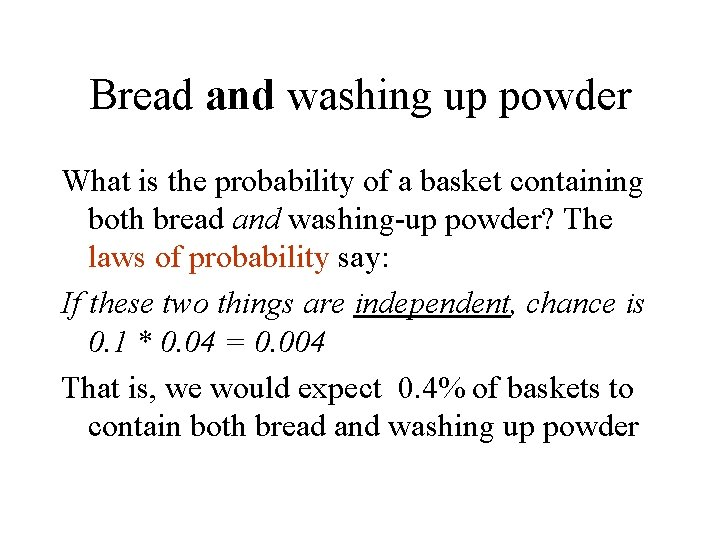Bread and washing up powder What is the probability of a basket containing both bread and washing-up powder? The laws of probability say: If these two things are independent, chance is 0. 1 * 0. 04 = 0. 004 That is, we would expect 0. 4% of baskets to contain both bread and washing up powder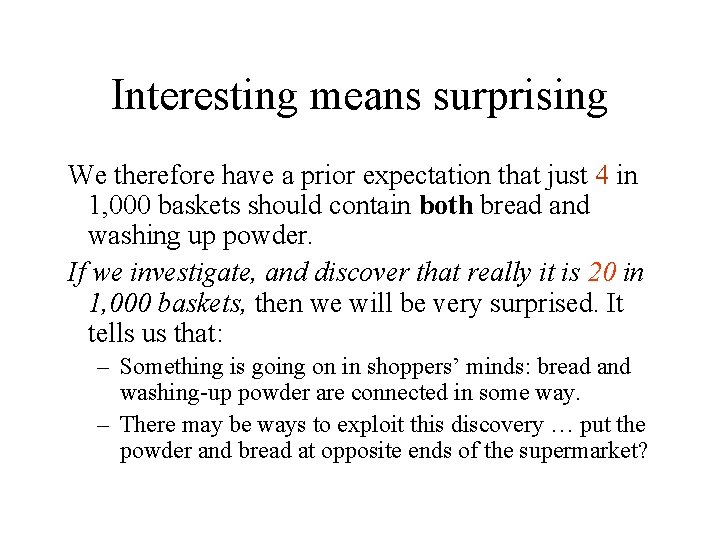Interesting means surprising We therefore have a prior expectation that just 4 in 1, 000 baskets should contain both bread and washing up powder. If we investigate, and discover that really it is 20 in 1, 000 baskets, then we will be very surprised. It tells us that: – Something is going on in shoppers’ minds: bread and washing-up powder are connected in some way. – There may be ways to exploit this discovery … put the powder and bread at opposite ends of the supermarket?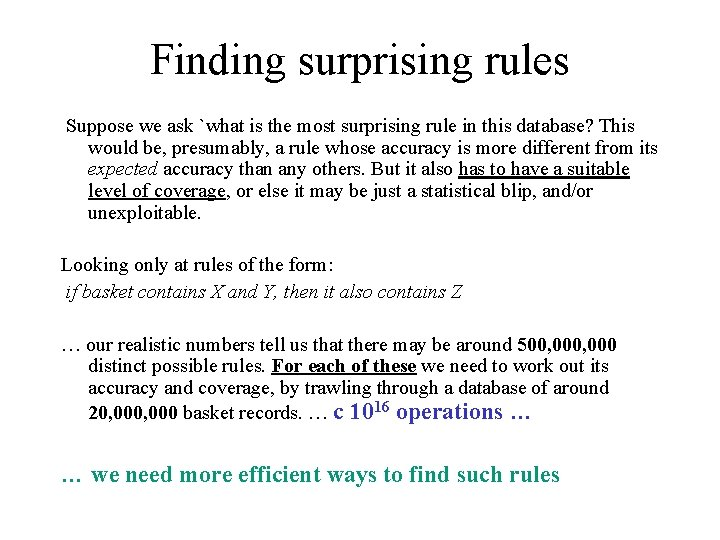Finding surprising rules Suppose we ask `what is the most surprising rule in this database? This would be, presumably, a rule whose accuracy is more different from its expected accuracy than any others. But it also has to have a suitable level of coverage, or else it may be just a statistical blip, and/or unexploitable. Looking only at rules of the form: if basket contains X and Y, then it also contains Z … our realistic numbers tell us that there may be around 500, 000 distinct possible rules. For each of these we need to work out its accuracy and coverage, by trawling through a database of around 20, 000 basket records. … c 1016 operations … … we need more efficient ways to find such rulesThe Apriori Algorithm There is nothing very special or clever about Apriori; but it is simple, fast, and very good at finding interesting rules of a specific kind in baskets or other transaction data, using operations that are efficient in standard database systems. It is used a lot in the R&D Depts of retailers in industry (or by consultancies who do work for them). But note that we will now talk about itemsets instead of rules. Also, the coverage of a rule is the same as the support of an itemset. Don’t get confused!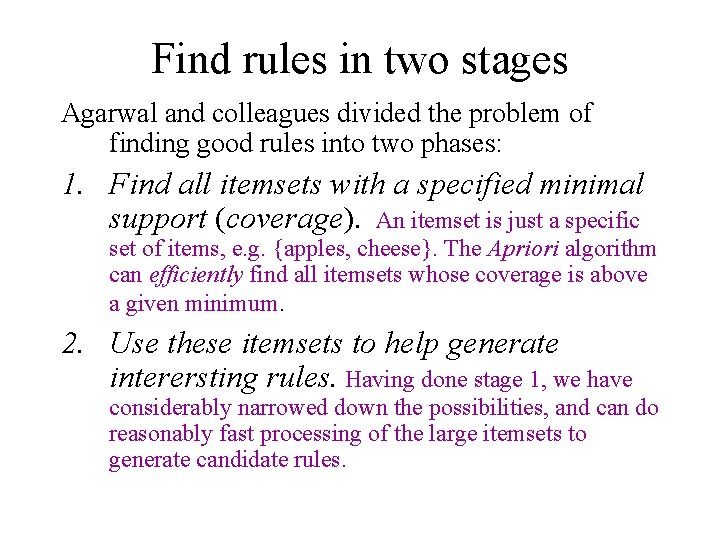Find rules in two stages Agarwal and colleagues divided the problem of finding good rules into two phases: 1. Find all itemsets with a specified minimal support (coverage). An itemset is just a specific set of items, e. g. {apples, cheese}. The Apriori algorithm can efficiently find all itemsets whose coverage is above a given minimum. 2. Use these itemsets to help generate interersting rules. Having done stage 1, we have considerably narrowed down the possibilities, and can do reasonably fast processing of the large itemsets to generate candidate rules.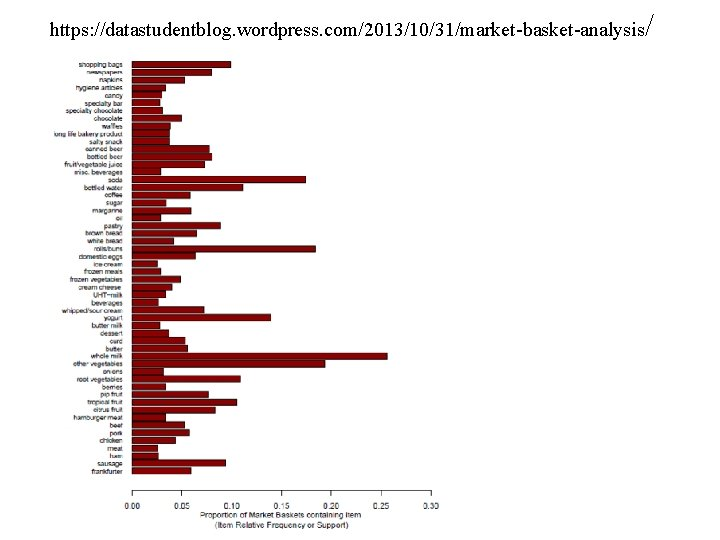Terminology k-itemset : a set of k items. E. g. {beer, cheese, eggs} is a 3 -itemset {cheese} is a 1 -itemset {honey, ice-cream} is a 2 -itemset support: an itemset has support s% if s% of the records in the DB contain that itemset. minimum support: the Apriori algorithm starts with the specification of a minimum level of support, and will focus on itemsets with this level or above.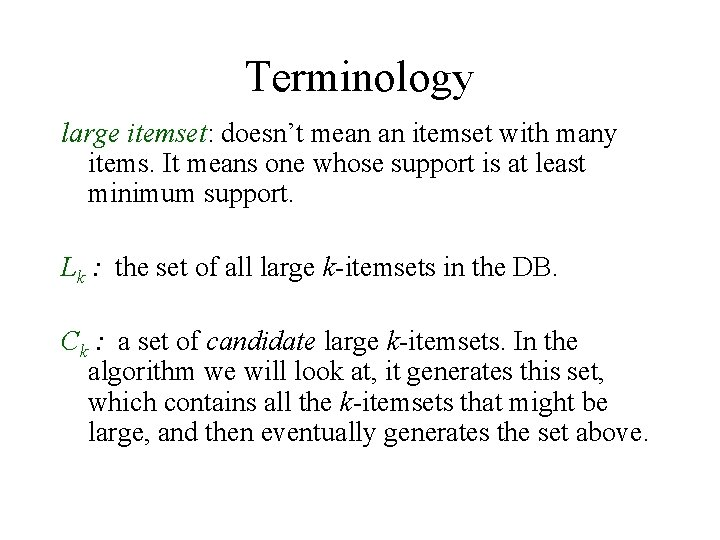Terminology large itemset: doesn’t mean an itemset with many items. It means one whose support is at least minimum support. Lk : the set of all large k-itemsets in the DB. Ck : a set of candidate large k-itemsets. In the algorithm we will look at, it generates this set, which contains all the k-itemsets that might be large, and then eventually generates the set above.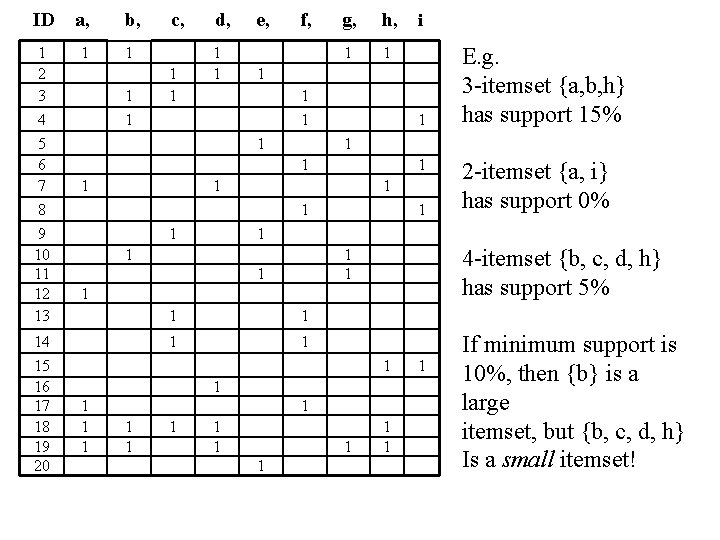ID a, b, 1 2 3 4 5 6 7 8 9 10 11 12 13 1 1 14 15 16 17 18 19 20 1 1 c, 1 1 d, 1 1 e, f, g, h, 1 1 1 1 4 -itemset {b, c, d, h} has support 5% 1 1 1 1 2 -itemset {a, i} has support 0% 1 1 1 E. g. 3 -itemset {a, b, h} has support 15% 1 1 1 i 1 1 1 1 If minimum support is 10%, then {b} is a large itemset, but {b, c, d, h} Is a small itemset!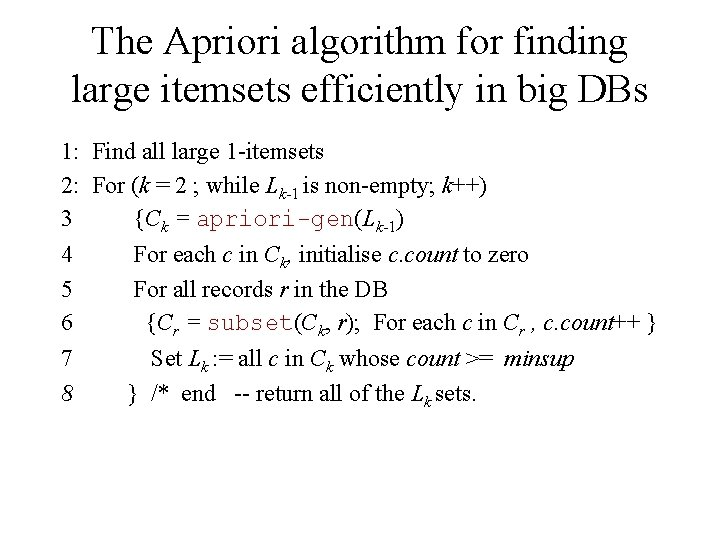The Apriori algorithm for finding large itemsets efficiently in big DBs 1: Find all large 1 -itemsets 2: For (k = 2 ; while Lk-1 is non-empty; k++) 3 {Ck = apriori-gen(Lk-1) 4 5 6 For each c in Ck, initialise c. count to zero For all records r in the DB {Cr = subset(Ck, r); For each c in Cr , c. count++ } 7 8 Set Lk : = all c in Ck whose count >= minsup } /* end -- return all of the Lk sets.The Apriori algorithm for finding large itemsets efficiently in big DBs 1: Find all large 1 -itemsets 2: For (k = 2 ; while Lk-1 is non-empty; k++) 3 {Ck = apriori-gen(L Generate candidate k-1) 2 -itemsets 4 5 6 For each c in Ck, initialise c. count to zero For all records r in the DB {Cr = subset(Ck, r); For each c in Cr , c. count++ } 7 8 } /* end -- return all of the Lk sets. Prune them to leave the valid ones Set Lk : = all c in Ck whose count >= minsup (those with enough support)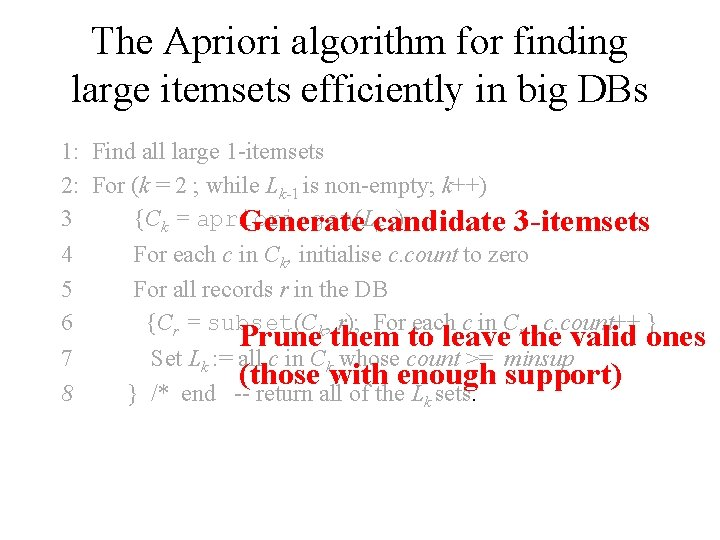The Apriori algorithm for finding large itemsets efficiently in big DBs 1: Find all large 1 -itemsets 2: For (k = 2 ; while Lk-1 is non-empty; k++) 3 {Ck = apriori-gen(L Generate candidate k-1) 3 -itemsets 4 5 6 For each c in Ck, initialise c. count to zero For all records r in the DB {Cr = subset(Ck, r); For each c in Cr , c. count++ } 7 8 } /* end -- return all of the Lk sets. Prune them to leave the valid ones Set Lk : = all c in Ck whose count >= minsup (those with enough support)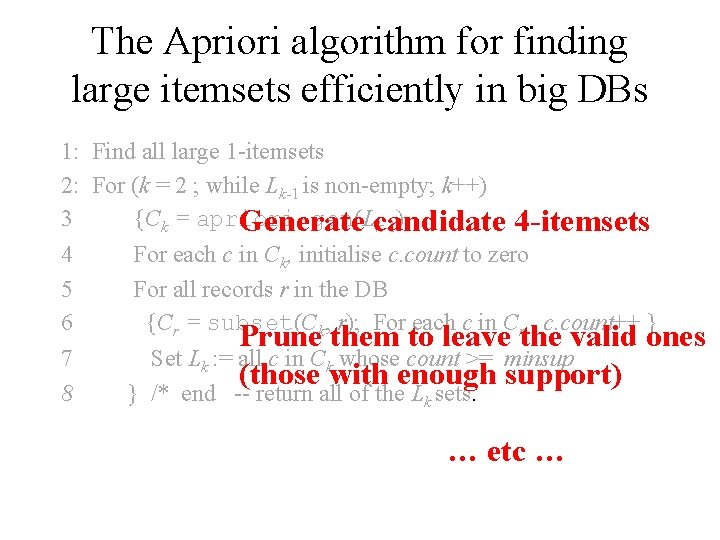The Apriori algorithm for finding large itemsets efficiently in big DBs 1: Find all large 1 -itemsets 2: For (k = 2 ; while Lk-1 is non-empty; k++) 3 {Ck = apriori-gen(L Generate candidate k-1) 4 -itemsets 4 5 6 For each c in Ck, initialise c. count to zero For all records r in the DB {Cr = subset(Ck, r); For each c in Cr , c. count++ } 7 8 } /* end -- return all of the Lk sets. Prune them to leave the valid ones Set Lk : = all c in Ck whose count >= minsup (those with enough support) … etc …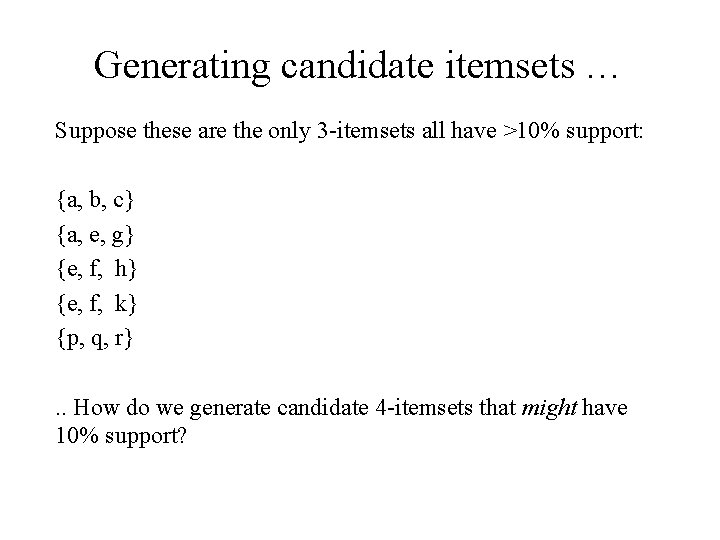Generating candidate itemsets … Suppose these are the only 3 -itemsets all have >10% support: {a, b, c} {a, e, g} {e, f, h} {e, f, k} {p, q, r}. . How do we generate candidate 4 -itemsets that might have 10% support?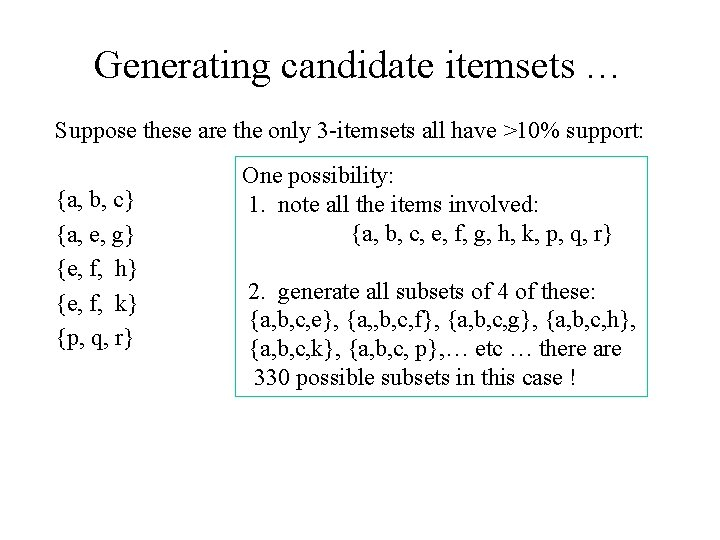Generating candidate itemsets … Suppose these are the only 3 -itemsets all have >10% support: {a, b, c} {a, e, g} {e, f, h} {e, f, k} {p, q, r} One possibility: 1. note all the items involved: {a, b, c, e, f, g, h, k, p, q, r} 2. generate all subsets of 4 of these: {a, b, c, e}, {a, , b, c, f}, {a, b, c, g}, {a, b, c, h}, {a, b, c, k}, {a, b, c, p}, … etc … there are 330 possible subsets in this case !Generating candidate itemsets … Suppose these are the only 3 -itemsets all have >10% support: {a, b, c} {a, e, g} {e, f, h} {e, f, k} {p, q, r} One possibility: 1. note all the items involved: {a, b, c, e, f, g, h, k, p, q, r} 2. generate all subsets of 4 of these: {a, b, c, e}, {a, b, c, f}, {a, b, c, g}, {a, b, c, h}, {a, b, c, k}, {a, b, c, p}, … etc … there are 330 possible subsets in this case ! But, hold on: we can easily see that {a, b, c, e} couldn’t have 10% support – because {a, b, e} is not one of our 3 -itsemsets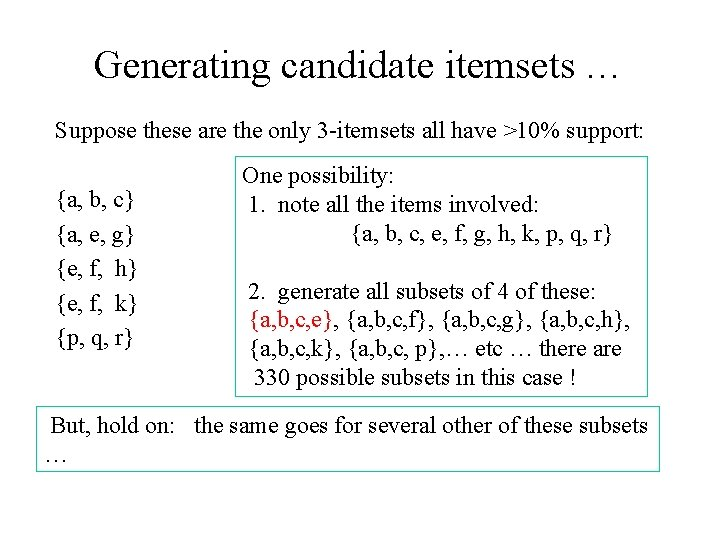Generating candidate itemsets … Suppose these are the only 3 -itemsets all have >10% support: {a, b, c} {a, e, g} {e, f, h} {e, f, k} {p, q, r} One possibility: 1. note all the items involved: {a, b, c, e, f, g, h, k, p, q, r} 2. generate all subsets of 4 of these: {a, b, c, e}, {a, b, c, f}, {a, b, c, g}, {a, b, c, h}, {a, b, c, k}, {a, b, c, p}, … etc … there are 330 possible subsets in this case ! But, hold on: the same goes for several other of these subsets …A neat Apriori trick {a, b, c} {a, e, g} {e, f, h} {e, f, k} {p, q, r} i. enforce that subsets are always arranged ‘lexicographically’ (or similar), as they are already on the left ii. Only generate k+1 -itemset candidates from k-itemsets that differ in the last item. So, in this case, the only candidate 4 -itemset would be: {e, f, h, k}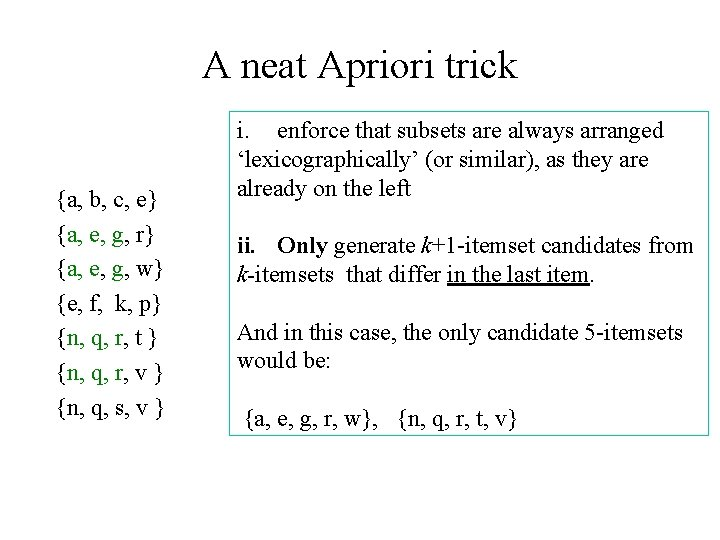A neat Apriori trick {a, b, c, e} {a, e, g, r} {a, e, g, w} {e, f, k, p} {n, q, r, t } {n, q, r, v } {n, q, s, v } i. enforce that subsets are always arranged ‘lexicographically’ (or similar), as they are already on the left ii. Only generate k+1 -itemset candidates from k-itemsets that differ in the last item. And in this case, the only candidate 5 -itemsets would be: {a, e, g, r, w}, {n, q, r, t, v}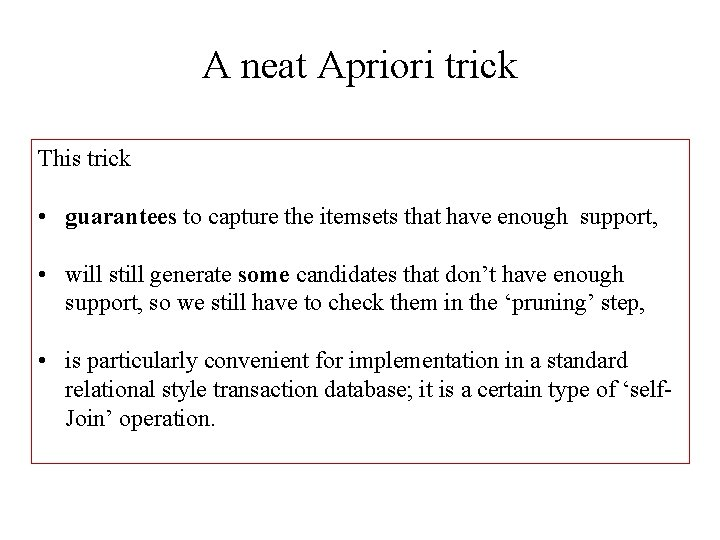A neat Apriori trick This trick • guarantees to capture the itemsets that have enough support, • will still generate some candidates that don’t have enough support, so we still have to check them in the ‘pruning’ step, • is particularly convenient for implementation in a standard relational style transaction database; it is a certain type of ‘self. Join’ operation.Explaining the Apriori Algorithm … 1: Find all large 1 -itemsets To start off, we simply find all of the large 1 -itemsets. This is done by a basic scan of the DB. We take each item in turn, and count the number of times that item appears in a basket. In our running example, suppose minimum support was 60%, then the only large 1 -itemsets would be: {a}, {b}, {c}, {d} and {f}. So we get L 1 = { {a}, {b}, {c}, {d}, {f}}Explaining the Apriori Algorithm … 1: Find all large 1 -itemsets 2: For (k = 2 ; while Lk-1 is non-empty; k++) We already have L 1. This next bit just means that the remainder of the algorithm generates L 2, L 3 , and so on until we get to an Lk that’s empty. How these are generated is like this: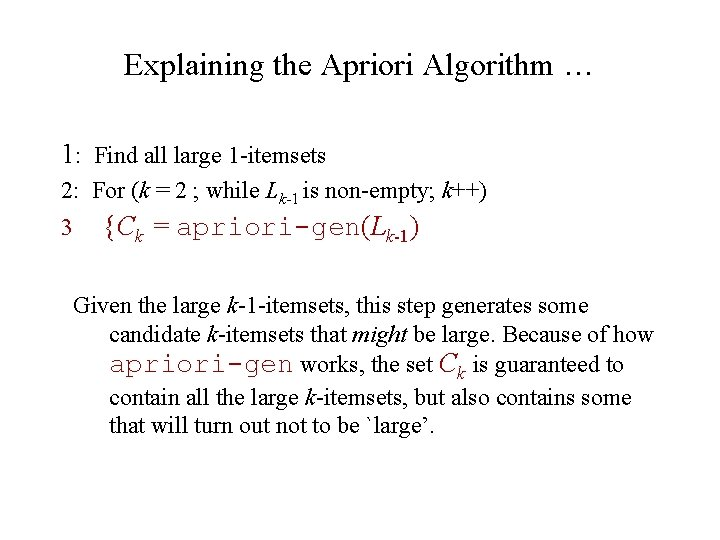Explaining the Apriori Algorithm … 1: Find all large 1 -itemsets 2: For (k = 2 ; while Lk-1 is non-empty; k++) {Ck = apriori-gen(Lk-1) 3 Given the large k-1 -itemsets, this step generates some candidate k-itemsets that might be large. Because of how apriori-gen works, the set Ck is guaranteed to contain all the large k-itemsets, but also contains some that will turn out not to be `large’.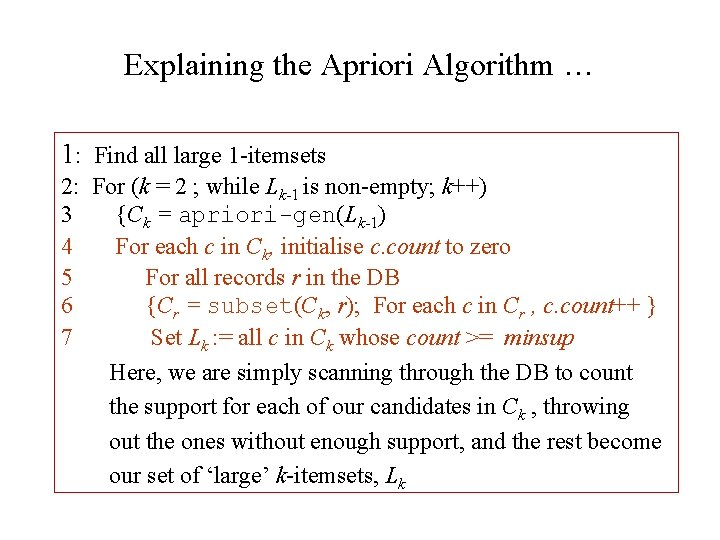Explaining the Apriori Algorithm … 1: Find all large 1 -itemsets 2: For (k = 2 ; while Lk-1 is non-empty; k++) 3 {Ck = apriori-gen(Lk-1) 4 For each c in Ck, initialise c. count to zero 5 For all records r in the DB 6 {Cr = subset(Ck, r); For each c in Cr , c. count++ } 7 Set Lk : = all c in Ck whose count >= minsup Here, we are simply scanning through the DB to count the support for each of our candidates in Ck , throwing out the ones without enough support, and the rest become our set of ‘large’ k-itemsets, LkExplaining the Apriori Algorithm … 1: Find all large 1 -itemsets 2: For (k = 2 ; while Lk-1 is non-empty; k++) 3 {Ck = apriori-gen(Lk-1) 4 For each c in Ck, initialise c. count to zero 5 For all records r in the DB 6 {Cr = subset(Ck, r); For each c in Cr , c. count++ } 7 Set Lk : = all c in Ck whose count >= minsup 8 } /* end -- return all of the Lk sets. We finish at the point where we get an empty Lk. The algorithm returns all of the (non-empty) Lk sets, from L 1 through to whichever was the last non-empty one. This gives us an excellent start in finding interesting rules (although the large itemsets themselves will usually be very interesting and useful).From itemsets to rules The Apriori algorithm finds interesting (i. e. frequent) itemsets. E. g. it may find that {apples, bananas, milk} has coverage 30% -- so 30% of transactions contain each of these three things. What can you say about the coverage of {apples, milk}? We can invent several potential rules, e. g. : IF basket contains apples and bananas, it also contains MILK. Suppose support of {a, b} is 40%; what is the confidence of this rule?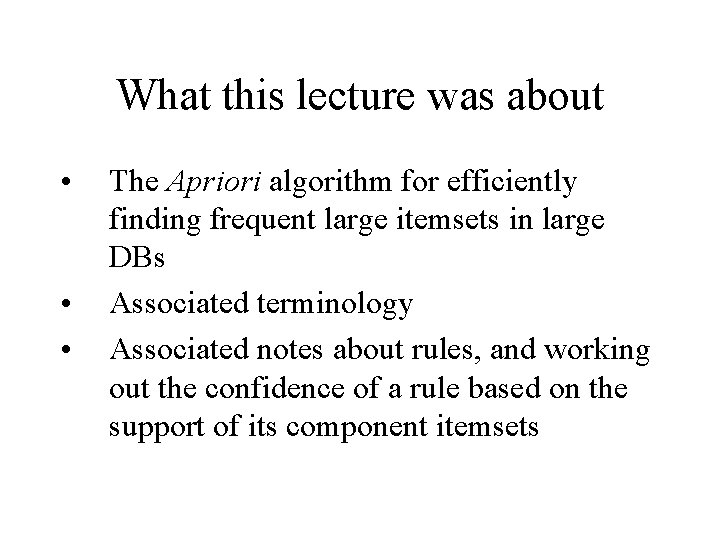What this lecture was about • • • The Apriori algorithm for efficiently finding frequent large itemsets in large DBs Associated terminology Associated notes about rules, and working out the confidence of a rule based on the support of its component itemsets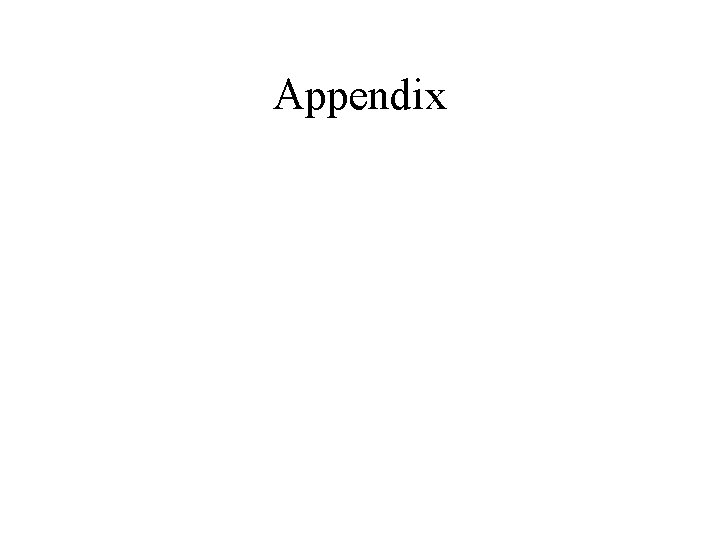Appendix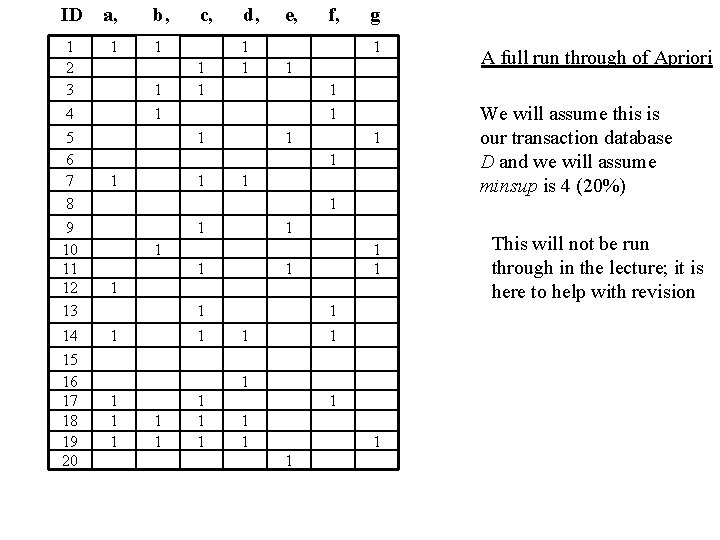ID a, b, 1 2 3 4 5 6 7 8 9 10 11 12 13 1 1 14 15 16 17 18 19 20 1 1 1 c, 1 1 d, 1 1 e, f, g 1 1 1 1 1 1 1 1 1 1 A full run through of Apriori We will assume this is our transaction database D and we will assume minsup is 4 (20%) This will not be run through in the lecture; it is here to help with revision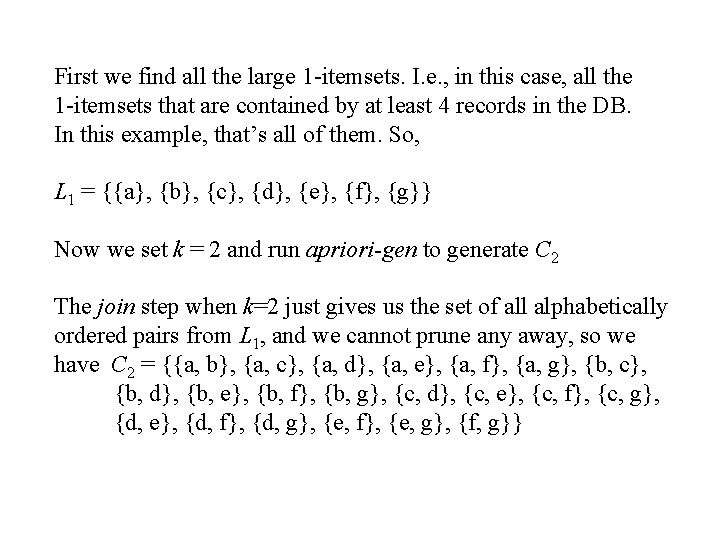First we find all the large 1 -itemsets. I. e. , in this case, all the 1 -itemsets that are contained by at least 4 records in the DB. In this example, that’s all of them. So, L 1 = {{a}, {b}, {c}, {d}, {e}, {f}, {g}} Now we set k = 2 and run apriori-gen to generate C 2 The join step when k=2 just gives us the set of all alphabetically ordered pairs from L 1, and we cannot prune any away, so we have C 2 = {{a, b}, {a, c}, {a, d}, {a, e}, {a, f}, {a, g}, {b, c}, {b, d}, {b, e}, {b, f}, {b, g}, {c, d}, {c, e}, {c, f}, {c, g}, {d, e}, {d, f}, {d, g}, {e, f}, {e, g}, {f, g}}So we have C 2 = {{a, b}, {a, c}, {a, d}, {a, e}, {a, f}, {a, g}, {b, c}, {b, d}, {b, e}, {b, f}, {b, g}, {c, d}, {c, e}, {c, f}, {c, g}, {d, e}, {d, f}, {d, g}, {e, f}, {e, g}, {f, g}} Line 4 of the Apriori algorithm now tells us set a counter for each of these to 0. Line 5 now prepares us to take each record in the DB in turn, and find which of those in C 2 are contained in it. The first record r 1 is: {a, b, d, g}. Those of C 2 it contains are: {a, b}, {a, d}, {a, g}, {b, d}, {b, g}, {d, g}. Hence Cr 1 = {{a, b}, {a, d}, {a, g}, {b, d}, {b, g}, {d, g}} and the rest of line 6 tells us to increment the counters of these itemsets. The second record r 2 is: {c, d, e}; Cr 2 = {{c, d}, {c, e}, {d, e}}, and we increment the counters for these three itemsets. … After all 20 records, we look at the counters, and in this case we will find that the itemsets with >= minsup (4) counters are: {a, d}, {c, e}. So, L 2 = {{a, c}, {a, d}, {c, e}, {c, f}}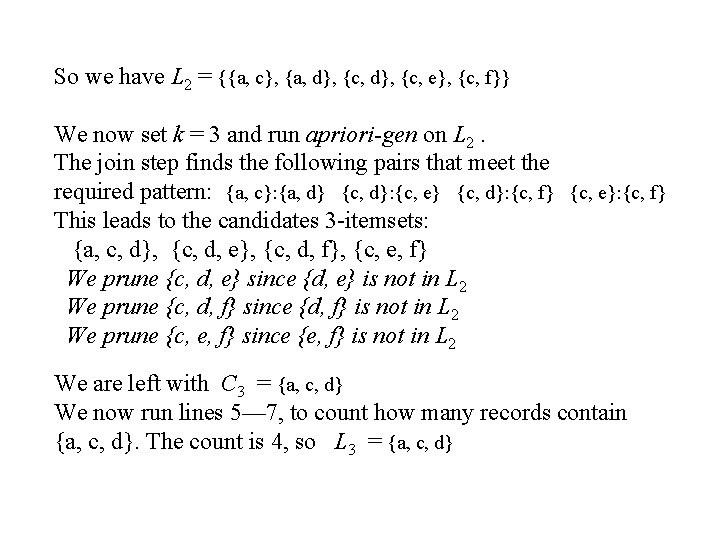So we have L 2 = {{a, c}, {a, d}, {c, e}, {c, f}} We now set k = 3 and run apriori-gen on L 2. The join step finds the following pairs that meet the required pattern: {a, c}: {a, d} {c, d}: {c, e} {c, d}: {c, f} {c, e}: {c, f} This leads to the candidates 3 -itemsets: {a, c, d}, {c, d, e}, {c, d, f}, {c, e, f} We prune {c, d, e} since {d, e} is not in L 2 We prune {c, d, f} since {d, f} is not in L 2 We prune {c, e, f} since {e, f} is not in L 2 We are left with C 3 = {a, c, d} We now run lines 5— 7, to count how many records contain {a, c, d}. The count is 4, so L 3 = {a, c, d}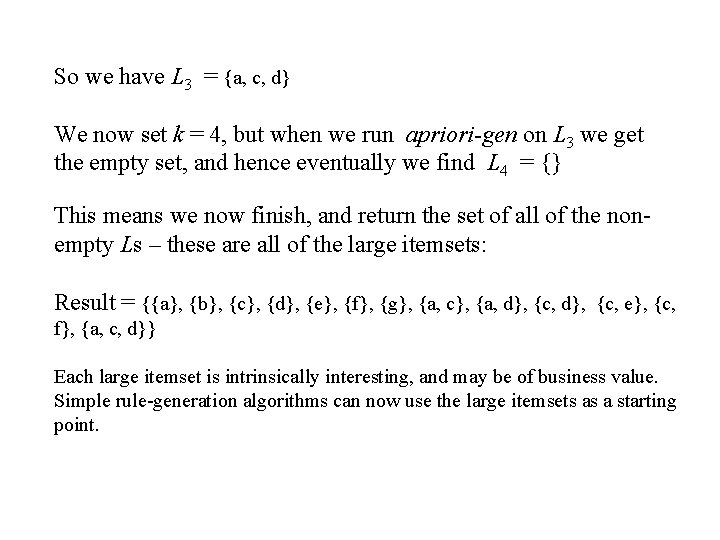So we have L 3 = {a, c, d} We now set k = 4, but when we run apriori-gen on L 3 we get the empty set, and hence eventually we find L 4 = {} This means we now finish, and return the set of all of the nonempty Ls – these are all of the large itemsets: Result = {{a}, {b}, {c}, {d}, {e}, {f}, {g}, {a, c}, {a, d}, {c, e}, {c, f}, {a, c, d}} Each large itemset is intrinsically interesting, and may be of business value. Simple rule-generation algorithms can now use the large itemsets as a starting point.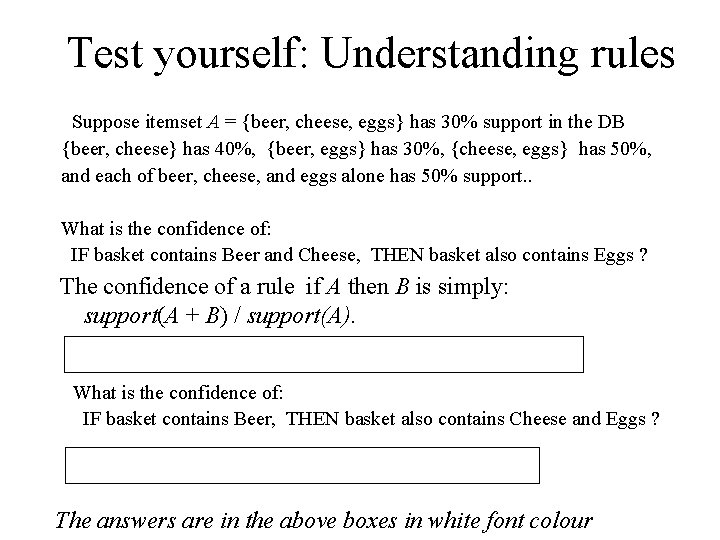Test yourself: Understanding rules Suppose itemset A = {beer, cheese, eggs} has 30% support in the DB {beer, cheese} has 40%, {beer, eggs} has 30%, {cheese, eggs} has 50%, and each of beer, cheese, and eggs alone has 50% support. . What is the confidence of: IF basket contains Beer and Cheese, THEN basket also contains Eggs ? The confidence of a rule if A then B is simply: support(A + B) / support(A). So it’s 30/40 = 0. 75 ; this rule has 75% confidence What is the confidence of: IF basket contains Beer, THEN basket also contains Cheese and Eggs ? 30 / 50 = 0. 6 so this rule has 60% confidence The answers are in the above boxes in white font colour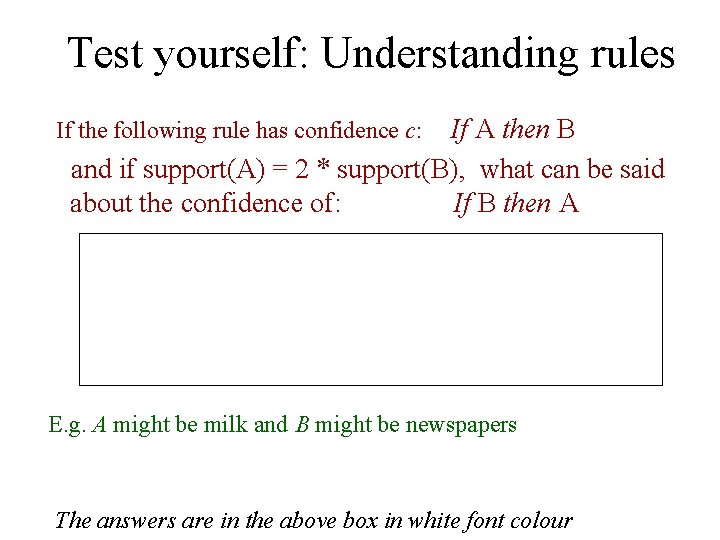Test yourself: Understanding rules If A then B and if support(A) = 2 * support(B), what can be said about the confidence of: If B then A If the following rule has confidence c: confidence c is support(A + B) / support(A) = support(A + B) / 2 * support(B) Let d be the confidence of ``If B then A’’. d is support(A+B / support(B) -- Clearly, d = 2 c E. g. A might be milk and B might be newspapers The answers are in the above box in white font colour